# Algorithms and Flowchart.ppt

13. Feb 2023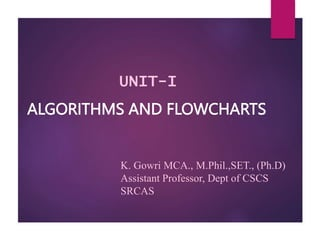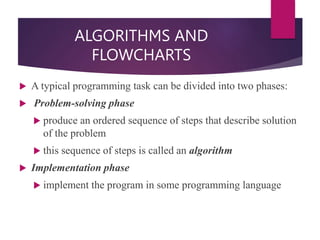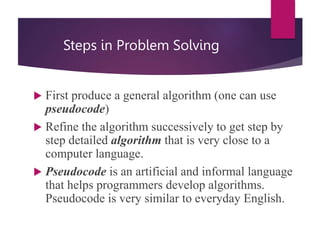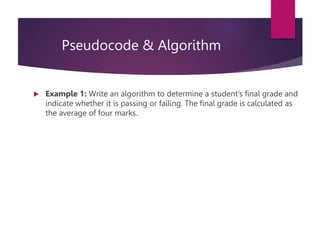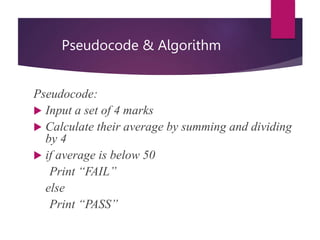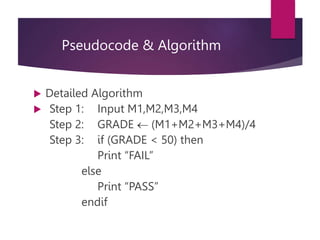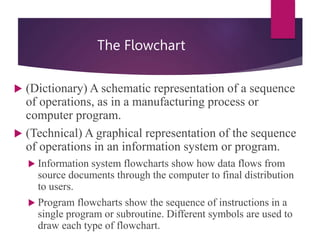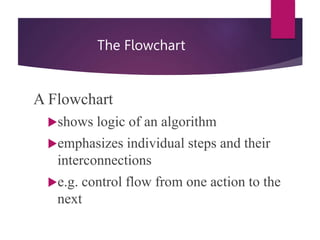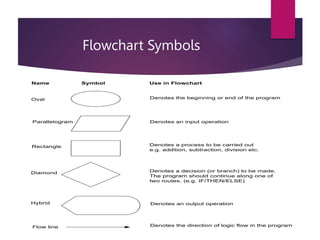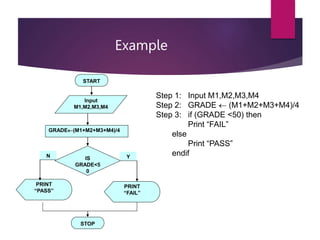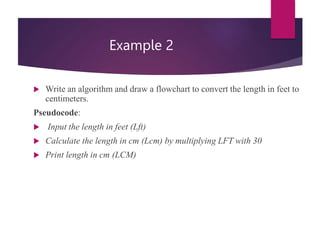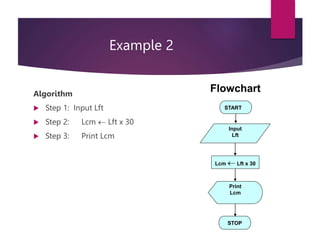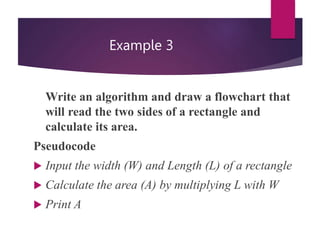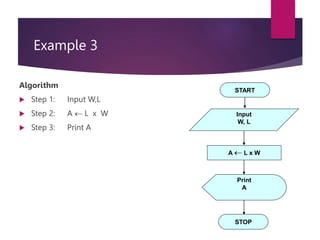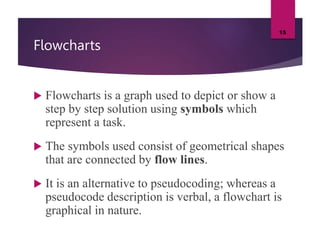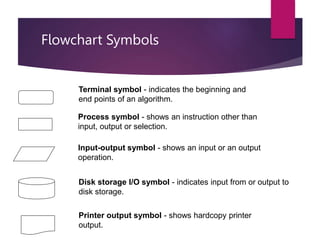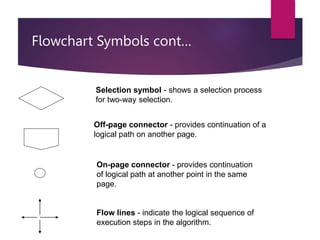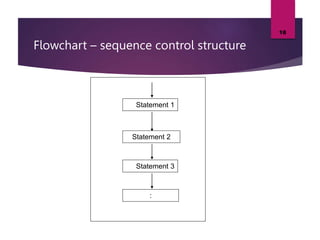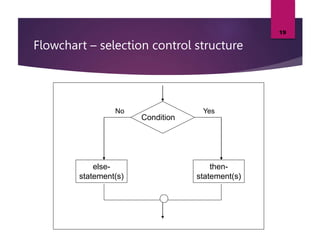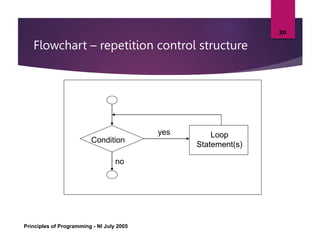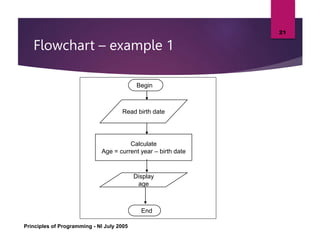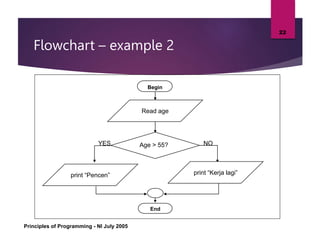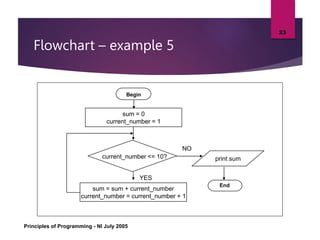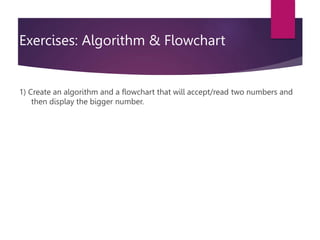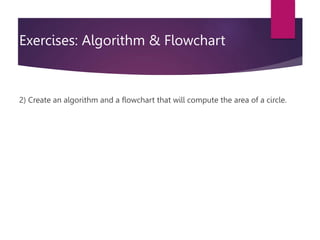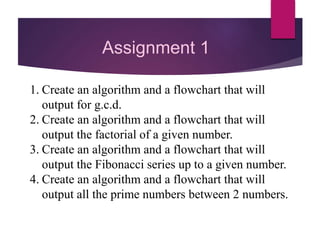1 von 26

### Algorithms and Flowchart.ppt

• 1. ALGORITHMS AND FLOWCHARTS K. Gowri MCA., M.Phil.,SET., (Ph.D) Assistant Professor, Dept of CSCS SRCAS UNIT-I
• 2. ALGORITHMS AND FLOWCHARTS  A typical programming task can be divided into two phases:  Problem-solving phase  produce an ordered sequence of steps that describe solution of the problem  this sequence of steps is called an algorithm  Implementation phase  implement the program in some programming language
• 3. Steps in Problem Solving  First produce a general algorithm (one can use pseudocode)  Refine the algorithm successively to get step by step detailed algorithm that is very close to a computer language.  Pseudocode is an artificial and informal language that helps programmers develop algorithms. Pseudocode is very similar to everyday English.
• 4. Pseudocode & Algorithm  Example 1: Write an algorithm to determine a student’s final grade and indicate whether it is passing or failing. The final grade is calculated as the average of four marks.
• 5. Pseudocode & Algorithm Pseudocode:  Input a set of 4 marks  Calculate their average by summing and dividing by 4  if average is below 50 Print “FAIL” else Print “PASS”
• 6. Pseudocode & Algorithm  Detailed Algorithm  Step 1: Input M1,M2,M3,M4 Step 2: GRADE  (M1+M2+M3+M4)/4 Step 3: if (GRADE < 50) then Print “FAIL” else Print “PASS” endif
• 7. The Flowchart  (Dictionary) A schematic representation of a sequence of operations, as in a manufacturing process or computer program.  (Technical) A graphical representation of the sequence of operations in an information system or program.  Information system flowcharts show how data flows from source documents through the computer to final distribution to users.  Program flowcharts show the sequence of instructions in a single program or subroutine. Different symbols are used to draw each type of flowchart.
• 8. The Flowchart A Flowchart shows logic of an algorithm emphasizes individual steps and their interconnections e.g. control flow from one action to the next
• 9. Flowchart Symbols Oval Parallelogram Rectangle Diamond Hybrid Name Symbol Use in Flowchart Denotes the beginning or end of the program Denotes an input operation Denotes an output operation Denotes a decision (or branch) to be made. The program should continue along one of two routes. (e.g. IF/THEN/ELSE) Denotes a process to be carried out e.g. addition, subtraction, division etc. Flow line Denotes the direction of logic flow in the program
• 10. Example PRINT “PASS” Step 1: Input M1,M2,M3,M4 Step 2: GRADE  (M1+M2+M3+M4)/4 Step 3: if (GRADE <50) then Print “FAIL” else Print “PASS” endif START Input M1,M2,M3,M4 GRADE(M1+M2+M3+M4)/4 IS GRADE<5 0 PRINT “FAIL” STOP Y N
• 11. Example 2  Write an algorithm and draw a flowchart to convert the length in feet to centimeters. Pseudocode:  Input the length in feet (Lft)  Calculate the length in cm (Lcm) by multiplying LFT with 30  Print length in cm (LCM)
• 12. Example 2 Algorithm  Step 1: Input Lft  Step 2: Lcm  Lft x 30  Step 3: Print Lcm START Input Lft Lcm  Lft x 30 Print Lcm STOP Flowchart
• 13. Example 3 Write an algorithm and draw a flowchart that will read the two sides of a rectangle and calculate its area. Pseudocode  Input the width (W) and Length (L) of a rectangle  Calculate the area (A) by multiplying L with W  Print A
• 14. Example 3 Algorithm  Step 1: Input W,L  Step 2: A  L x W  Step 3: Print A START Input W, L A  L x W Print A STOP
• 15. Flowcharts  Flowcharts is a graph used to depict or show a step by step solution using symbols which represent a task.  The symbols used consist of geometrical shapes that are connected by flow lines.  It is an alternative to pseudocoding; whereas a pseudocode description is verbal, a flowchart is graphical in nature. 15
• 16. Flowchart Symbols Terminal symbol - indicates the beginning and end points of an algorithm. Process symbol - shows an instruction other than input, output or selection. Input-output symbol - shows an input or an output operation. Disk storage I/O symbol - indicates input from or output to disk storage. Printer output symbol - shows hardcopy printer output.
• 17. Flowchart Symbols cont… Selection symbol - shows a selection process for two-way selection. Off-page connector - provides continuation of a logical path on another page. On-page connector - provides continuation of logical path at another point in the same page. Flow lines - indicate the logical sequence of execution steps in the algorithm.
• 18. Flowchart – sequence control structure 18 Statement 2 Statement 1 Statement 3 :
• 19. Flowchart – selection control structure 19 Condition else- statement(s) then- statement(s) Yes No
• 20. Flowchart – repetition control structure Principles of Programming - NI July 2005 20 Condition Loop Statement(s) yes no
• 21. Flowchart – example 1 Principles of Programming - NI July 2005 21 Begin Read birth date Calculate Age = current year – birth date Display age End
• 22. Flowchart – example 2 Principles of Programming - NI July 2005 22 Begin Read age End Age > 55? NO YES print “Pencen” print “Kerja lagi”
• 23. Flowchart – example 5 Principles of Programming - NI July 2005 23 Begin End current_number <= 10? NO YES sum = 0 current_number = 1 sum = sum + current_number current_number = current_number + 1 print sum
• 24. Exercises: Algorithm & Flowchart 1) Create an algorithm and a flowchart that will accept/read two numbers and then display the bigger number.
• 25. Exercises: Algorithm & Flowchart 2) Create an algorithm and a flowchart that will compute the area of a circle.
• 26. Assignment 1 1. Create an algorithm and a flowchart that will output for g.c.d. 2. Create an algorithm and a flowchart that will output the factorial of a given number. 3. Create an algorithm and a flowchart that will output the Fibonacci series up to a given number. 4. Create an algorithm and a flowchart that will output all the prime numbers between 2 numbers.